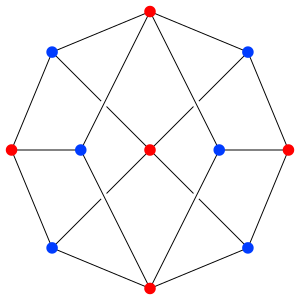# Hamiltonian path facts for kids

Kids Encyclopedia FactsThe Herschel graph is the smallest possible polyhedral graph that does not have a Hamiltonian cycle.

A Hamiltonian path is a path in a graph which contains each vertex of the graph exactly once. A Hamiltonian cycle is a Hamiltonian path, which is also a cycle. Knowing whether such a path exists in a graph, as well as finding it is a fundamental problem of graph theory. It is much more difficult than finding an Eulerian path, which contains each edge exactly once. The problem of finding a Hamiltonian path is NP-complete. There are two classes of graphs: directed and undirected graphs. In directed graphs, an edge can only be travelled in one direction, in undirected graphs it can be travelled in both directions. The Travelling Salesman Problem is an instance of this problem. Its task is to find the shortest Hamiltonian cycle, usually in a directed graph, with weight (or distances) attached to the edges.

Hamiltonian paths and cycles and cycle paths are named after William Rowan Hamilton who invented the Icosian game. This game is also known as Hamilton's puzzle. It involves finding a Hamiltonian cycle in the edge graph of the dodecahedron. Hamilton solved this problem using the Icosian Calculus, an algebraic structure based on roots of unity with many similarities to the quaternions (also invented by Hamilton). This solution does not generalize to arbitrary graphs. However, despite being named after Hamilton, Hamiltonian cycles in polyhedra had also been studied a year earlier by Thomas Kirkman.Hamiltonian path Facts for Kids. Kiddle Encyclopedia.